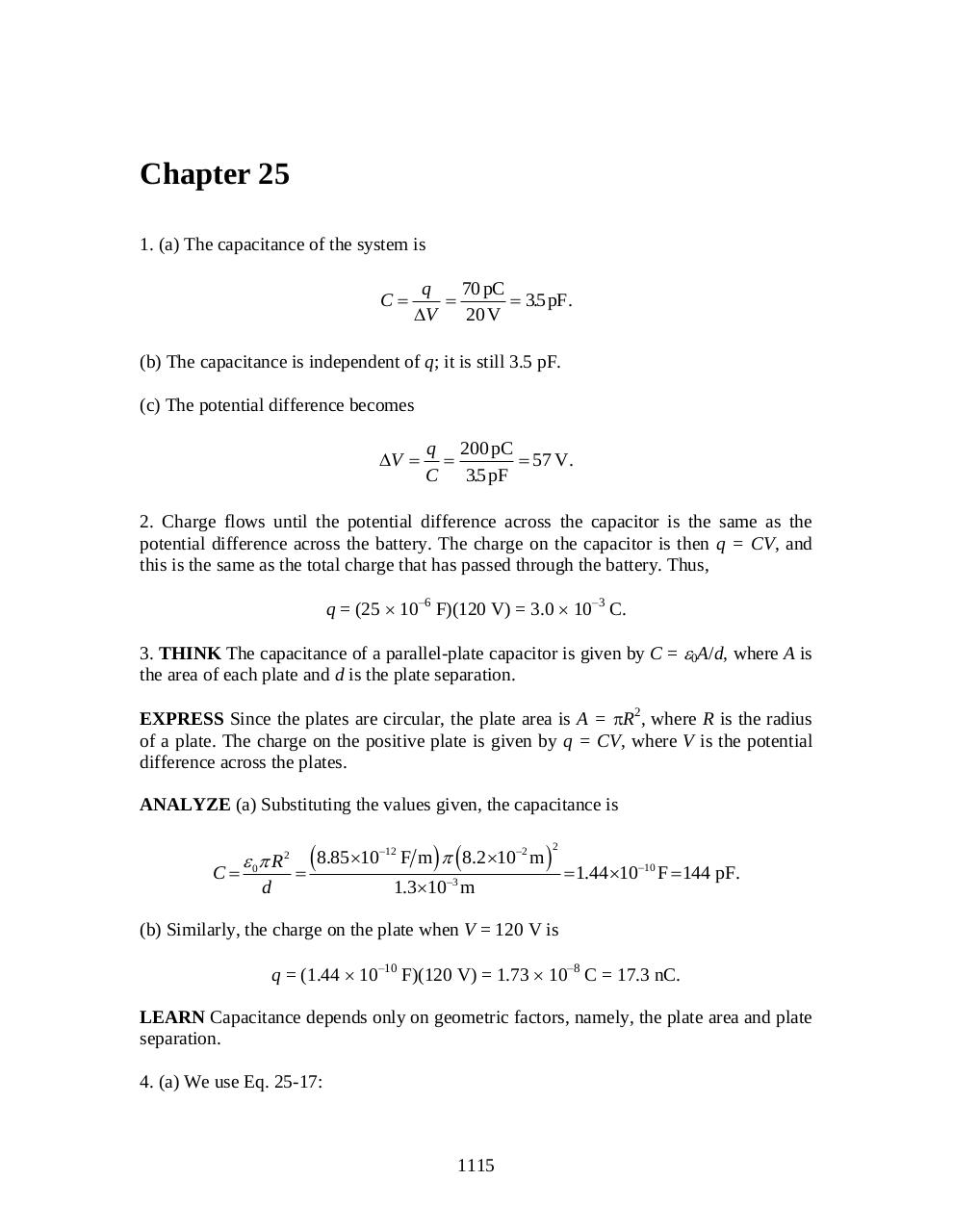# chapter 25.pdfPage 12332

#### Text preview

Chapter 25
1. (a) The capacitance of the system is
C

q
70 pC

 35
. pF.
V
20 V

(b) The capacitance is independent of q; it is still 3.5 pF.
(c) The potential difference becomes
V 

q 200 pC

 57 V.
C 35
. pF

2. Charge flows until the potential difference across the capacitor is the same as the
potential difference across the battery. The charge on the capacitor is then q = CV, and
this is the same as the total charge that has passed through the battery. Thus,
q = (25  10–6 F)(120 V) = 3.0  10–3 C.
3. THINK The capacitance of a parallel-plate capacitor is given by C = 0A/d, where A is
the area of each plate and d is the plate separation.
EXPRESS Since the plates are circular, the plate area is A = R2, where R is the radius
of a plate. The charge on the positive plate is given by q = CV, where V is the potential
difference across the plates.
ANALYZE (a) Substituting the values given, the capacitance is

C

 0 R 2
d

8.8510

12

F m   8.2 102 m 

2

3

1.310 m

 1.44 1010 F  144 pF.

(b) Similarly, the charge on the plate when V = 120 V is
q = (1.44  10–10 F)(120 V) = 1.73  10–8 C = 17.3 nC.
LEARN Capacitance depends only on geometric factors, namely, the plate area and plate
separation.
4. (a) We use Eq. 25-17:

1115